# SUPERPOSITION IN AC CIRCUITSClick or Tap the Example circuits below to invoke TINACloud and select the Interactive DC mode to Analyze them Online.
Get a low cost access to TINACloud to edit the examples or create your own circuitsVOLTAGE AND CURRENT DIVISION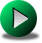THĂ‰VENIN AND NORTON EQUIVALENT CIRCUITS

We have already studied the superposition theorem for DC circuits. In this chapter we will show its application for AC circuits.

The superposition theorem states that in a linear circuit with several sources, the current and voltage for any element in the circuit is the sum of the currents and voltages produced by each source acting independently. The theorem is valid for any linear circuit. The best way to use superposition with AC circuits is to calculate the complex effective or peak value of the contribution of each source applied one at a time, and then to add the complex values. This is much easier than using superposition with time functions, where one has to add the individual time functions.

To calculate the contribution of each source independently, all the other sources must be removed and replaced without affecting the final result.

When removing a voltage source, its voltage must be set to zero, which is equivalent to replacing the voltage source with a short circuit.

When removing a current source, its current must be set to zero, which is equivalent to replacing the current source with an open circuit.

Now let's explore an example.

In the circuit shown below"

Ri = 100 ohm, R1 = 20 ohm, R2 = 12 ohm, L = 10 uH, C = 0.3 nF, vS(t)=50cos(wt) V, iS(t)=1cos(wt+30Â°) A, f=400 kHz.

Notice that both sources have the same frequency: we will only work in this chapter with sources all having the same frequency. Otherwise, superposition must be handled differently.

Find the currents i(t) and i1(t) using the superposition theorem.

Click/tap the circuit above to analyze on-line or click this link to Save under Windows

Let's use TINA and hand calculations in parallel to solve the problem.

First substitute an open circuit for the current source and calculate the complex phasors I', I1' due to the contribution only from VS.

The currents in this case are equal:

I' = I1' = VS/(Ri + R1 + j*w*L) = 50/(120+j2*p*4*105*10-5) = 0.3992-j0.0836

I' = 0.408 ej 11.83Â° A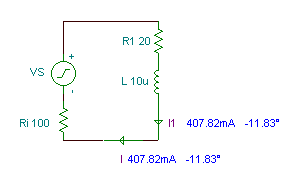Next substitute a short-circuit for the voltage source and calculate the complex phasors I'', I1'' due to the contribution only from IS.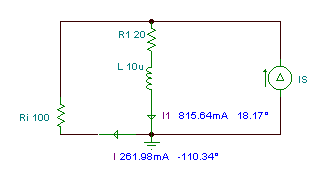In this case we can use the current division formula: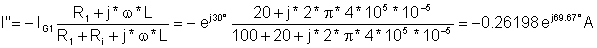I'' = -0.091 - j 0.246 A

and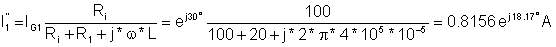I1" = 0.7749 + j 0.2545 A

The sum of the two steps:

I = I' + I" = 0.3082 - j 0.3286 = 0.451 e- j 46.9Â° A

I1 = I1" + I1' = 1.174 + j 0.1709 = 1.1865 ej 8.28Â° A

These results correspond well with the values calculated by TINA: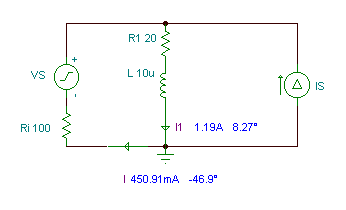The time functions of the currents:

i(t) = 0.451 cos (wĂ—t - 46.9Â°) A

i1(t) = 1.1865 cos (wĂ—t + 8.3Â°) A

Similarly, the results given by TINA's Interpreter also agree:

 {Solution by TINA's Interpreter} f:=400000; Vs:=50; IG:=1*exp(j*pi/6); om:=2*pi*f; sys I,I1 I+IG=I1 Vs=I*Ri+I1*(R1+j*om*L) end; I=[308.093m-329.2401m*j] abs(I)=[450.9106m] radtodeg(arc(I))=[-46.9004] abs(I1)=[1.1865] radtodeg(arc(I1))=[8.2749]

As we said in the DC chapter on superposition, it gets pretty complicated using the superposition theorem for circuits containing more then two sources. While the superposition theorem can be useful for solving simple practical problems, its main use is in the theory of circuit analysis, where it is employed in proving other theorems.VOLTAGE AND CURRENT DIVISIONTHĂ‰VENIN AND NORTON EQUIVALENT CIRCUITS